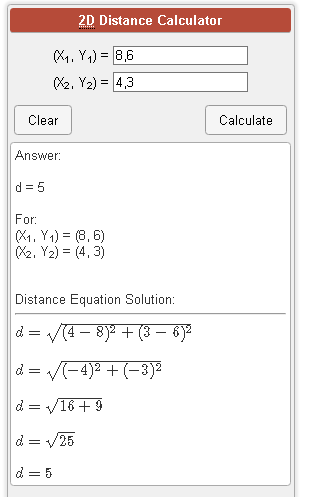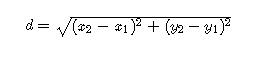DISTANCE

2D Distance Calculator (calculatorsoup.com)## Distance Formula

The distance between two points is the length of the path connecting them. The shortest path distance is a straight line. In a 2 dimensional plane, the distance between points (X1, Y1) and (X2, Y2) is given by the Pythagorean theorem: